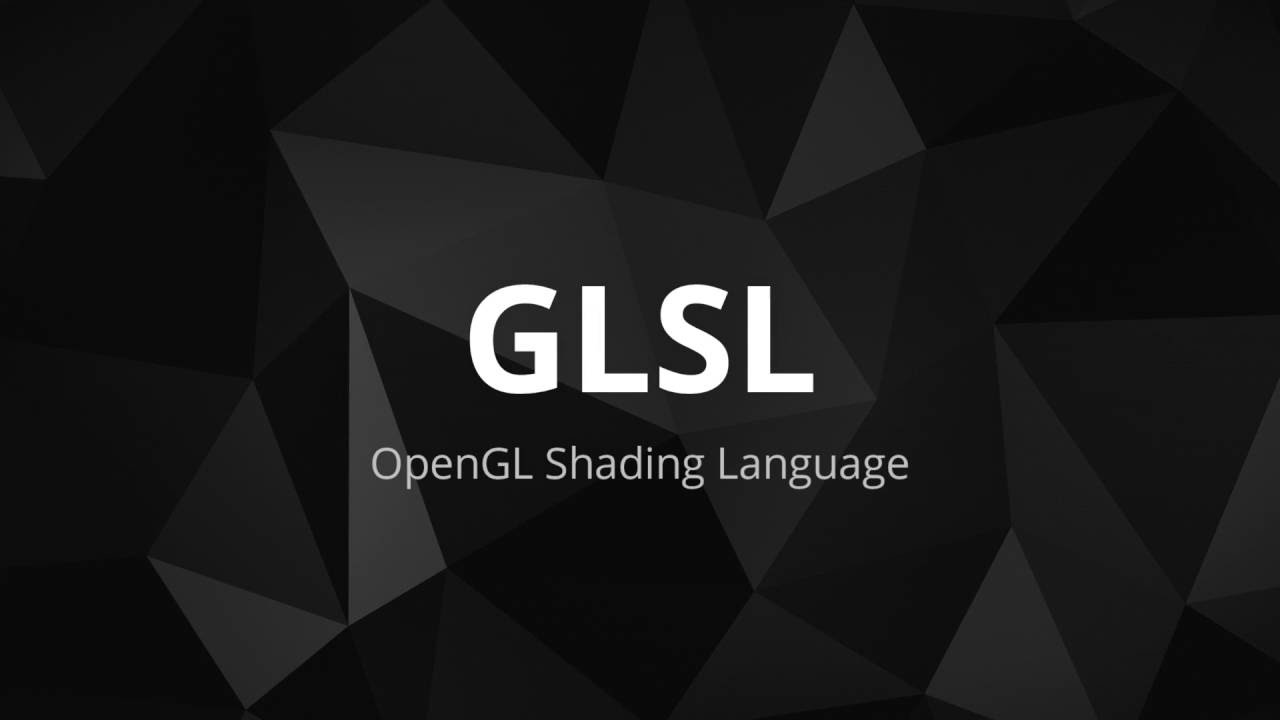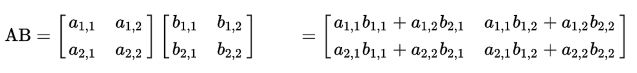# 初探 GLSL 着色器（二）### GLSL 中的运算

``````int a = 2.0;
vec4 v4=vec4(1.0, 1.0, 2, 1.0);
``````

GLSL 中的运算符与 C 语言的大致相似，但由于存在 向量 和 矩阵 这两种基本类型，所以还是有一些不同的。见下表：

1 () 聚组:a*(b+c) N/A
2 [] () . ++ -- 数组下标[],方法参数fun(arg1,arg2,arg3),属性访问a.b,自增/减后缀a++ a-- L - R
3 ++ -- + - ! 自增/减前缀++a --a,正负号(一般正号不写)a ,-a,取反!false R - L
4 * / 乘除数学运算 L - R
5 + - 加减数学运算 L - R
7 < > <= >= 关系运算符 L - R
8 == != 相等性运算符 L - R
12 && 逻辑与 L - R
13 ^^ 逻辑排他或(用处基本等于!=) L - R
14 || 逻辑或 L - R
15 ? : 三目运算符 L - R
16 = += -= *= /= 赋值与复合赋值 L - R
17 , 顺序分配运算 L - R

``````左值:表示一个储存位置,可以是变量,也可以是表达式,但表达式最后的结果必须是一个储存位置.

1.同类型 float int 间运算：

``float与float , int与int之间可以直接运算。``

2.不同类型 float int 间运算:

``````float 与 int 之间进行运算，需要进行一次显示转换.即要么把float转成int: int(1.0) ,要么把int转成float: float(1) ,以下表达式都是正确的:

```
int a=int(2.0);
float a= float(2);

int a=int(2.0)*2 + 1;
float a= float(2)*6.0+2.3;
`````````

3.float 与 vec(向量) mat(矩阵) 运算:

``````vec,mat这些类型其实是由float复合而成的,当它们与float运算时,其实就是在每一个分量上分别与float进行运算,这就是所谓的逐分量运算.glsl里 大部分涉及vec,mat的运算都是逐分量运算,但也并不全是. 下文中就会讲到特例.

int 与 vec,mat之间是不可运算的, 因为vec和mat中的每一个分量都是 float 类型的. 无法与int进行逐分量计算.

```
vec3 a = vec3(1.0, 2.0, 3.0);
mat3 m = mat3(1.0);
float s = 10.0;
vec3 b = s * a; // vec3(10.0, 20.0, 30.0)
vec3 c = a * s; // vec3(10.0, 20.0, 30.0)
mat3 m2 = s * m; // = mat3(10.0)
mat3 m3 = m * s; // = mat3(10.0)
`````````

4.vec(向量) 与 vec(向量):

``````两向量间的运算首先要保证操作数的阶数都相同.否则不能计算.例如: vec3*vec2 vec4+vec3 等等都是不行的.

```

vec3 a = vec3(1.0, 2.0, 3.0);
vec3 b = vec3(0.1, 0.2, 0.3);
vec3 c = a + b; // = vec3(1.1, 2.2, 3.3);
vec3 d = a * b; // = vec3(0.1, 0.4, 0.9);

`````````5.vec(向量) 与 mat(矩阵):

``````要保证操作数的阶数相同,且vec与mat间只存在乘法运算.

```
vec2 v = vec2(10., 20.);
mat2 m = mat2(1., 2.,  3., 4.);
vec2 w = m * v; // = vec2(1. * 10. + 3. * 20., 2. * 10. + 4. * 20.)
...

vec2 v = vec2(10., 20.);
mat2 m = mat2(1., 2.,  3., 4.);
vec2 w = v * m; // = vec2(1. * 10. + 2. * 20., 3. * 10. + 4. * 20.)
```

6.mat(矩阵) 与 mat(矩阵):

``````要保证操作数的阶数相同.

```
mat2 a = mat2(1., 2.,  3., 4.);
mat2 b = mat2(10., 20.,  30., 40.);
mat2 c = a * b; //mat2(1.*10.+3.*20.,2.*10.+4.*20.,1.* 30.+3.*40.,2.* 30.+4.*40.);

mat2 d = a+b;//mat2(1.+10.,2.+20.,3.+30.,4.+40);
```

### 类型转换

GLSL 可以通过构造函数进行显式转换，方法如下：

``````bool t= true;
bool f = false;

int a = int(t); //true转换为1或1.0
int a1 = int(f);//false转换为0或0.0

float b = float(t);
float b1 = float(f);

bool c = bool(0);//0或0.0转换为false
bool c1 = bool(1);//非0转换为true

bool d = bool(0.0);
bool d1 = bool(1.0);
``````

### 精度限定

glsl在进行光栅化着色的时候,会产生大量的浮点数运算,这些运算可能是当前设备所不能承受的,所以glsl提供了3种浮点数精度,我们可以根据不同的设备来使用合适的精度.

``````lowp float color;
varying mediump vec2 Coord;
lowp ivec2 foo(lowp mat3);
highp mat4 m;
``````

``````uniform highp float h1;
highp float h2 = 2.3 * 4.7; //运算过程和结果都 是高精度
mediump float m;
m = 3.7 * h1 * h2; //运算过程 是高精度
h2 = m * h1; //运算过程 是高精度
m = h2 – h1; //运算过程 是高精度
h2 = m + m; //运算过程和结果都 是中等精度
void f(highp float p); // 形参 p 是高精度
f(3.3); //传入的 3.3是高精度``````

invariant关键字:

``````#pragma STDGL invariant(all) //所有输出变量为 invariant
invariant varying texCoord; //varying在传递数据的时候声明为invariant``````

1.在一般变量中: invariant > storage > precision

2.在参数中: storage > parameter > precision

``````invariant varying lowp float color; // invariant > storage > precision

void doubleSize(const in lowp float s){ //storage > parameter > precision
float s1=s;
}``````

### 内置函数库

glsl提供了非常丰富的函数库,供我们使用,这些功能都是非常有用且会经常用到的. 这些函数按功能区分大改可以分成7类:

T abs(T x) 返回x的绝对值
T sign(T x) 比较x与0的值,大于,等于,小于 分别返回 1.0 ,0.0,-1.0
T floor(T x) 返回<=x的最大整数
T ceil(T x) 返回>=等于x的最小整数
T fract(T x) 获取x的小数部分
T mod(T x, T y)
T mod(T x, float y)

T min(T x, T y)
T min(T x, float y)

T max(T x, T y)
T max(T x, float y)

T clamp(T x, T minVal, T maxVal)
T clamp(T x, float minVal,float maxVal)
min(max(x, minVal), maxVal),返回值被限定在 minVal,maxVal之间
T mix(T x, T y, T a)
T mix(T x, T y, float a)

T step(T edge, T x)
T step(float edge, T x)

T smoothstep(T edge0, T edge1, T x)
T smoothstep(float edge0,float edge1, T x)

T sin(T angle) 正弦函数,角度是弧度
T cos(T angle) 余弦函数,角度是弧度
T tan(T angle) 正切函数,角度是弧度
T asin(T x) 反正弦函数,返回值是弧度
T acos(T x) 反余弦函数,返回值是弧度
T atan(T y, T x)
T atan(T y_over_x)

T pow(T x, T y) 返回x的y次幂 xy
T exp(T x) 返回x的自然指数幂 ex
T log(T x) 返回x的自然对数 ln
T exp2(T x) 返回2的x次幂 2x
T log2(T x) 返回2为底的对数 log2
T sqrt(T x) 开根号 √x
T inversesqrt(T x) 先开根号,在取倒数,就是 1/√x

float length(T x) 返回矢量x的长度
float distance(T p0, T p1) 返回p0 p1两点的距离
float dot(T x, T y) 返回x y的点积
vec3 cross(vec3 x, vec3 y) 返回x y的叉积
T normalize(T x) 对x进行归一化,保持向量方向不变但长度变为1
T faceforward(T N, T I, T Nref) 根据 矢量 N 与Nref 调整法向量
T reflect(T I, T N) 返回 I - 2 dot(N,I) N, 结果是入射矢量 I 关于法向量N的 镜面反射矢量
T refract(T I, T N, float eta) 返回入射矢量I关于法向量N的折射矢量,折射率为eta

mat可以为任意类型矩阵.

mat matrixCompMult(mat x, mat y) 将矩阵 x 和 y的元素逐分量相乘

bvec指的是由bool类型组成的一个向量:

``````vec3 v3= vec3(0.,0.,0.);
vec3 v3_1= vec3(1.,1.,1.);
bvec3 aa= lessThan(v3,v3_1); //bvec3(true,true,true)
``````

bvec lessThan(T x, T y) 逐分量比较x < y,将结果写入bvec对应位置
bvec lessThanEqual(T x, T y) 逐分量比较 x <= y,将结果写入bvec对应位置
bvec greaterThan(T x, T y) 逐分量比较 x > y,将结果写入bvec对应位置
bvec greaterThanEqual(T x, T y) 逐分量比较 x >= y,将结果写入bvec对应位置
bvec equal(T x, T y)
bvec equal(bvec x, bvec y)

bvec notEqual(T x, T y)
bvec notEqual(bvec x, bvec y)

bool any(bvec x) 如果x的任意一个分量是true,则结果为true
bool all(bvec x) 如果x的所有分量是true,则结果为true
bvec not(bvec x) bool矢量的逐分量取反

``````vec4 texture2DLod(sampler2D sampler, vec2 coord, float lod);
vec4 texture2DProjLod(sampler2D sampler, vec3 coord, float lod);
vec4 texture2DProjLod(sampler2D sampler, vec4 coord, float lod);
vec4 textureCubeLod(samplerCube sampler, vec3 coord, float lod);
``````

``````vec4 texture2D(sampler2D sampler, vec2 coord, float bias);
vec4 texture2DProj(sampler2D sampler, vec3 coord, float bias);
vec4 texture2DProj(sampler2D sampler, vec4 coord, float bias);
vec4 textureCube(samplerCube sampler, vec3 coord, float bias);``````

``````vec4 texture2D(sampler2D sampler, vec2 coord);
vec4 texture2DProj(sampler2D sampler, vec3 coord);
vec4 texture2DProj(sampler2D sampler, vec4 coord);
vec4 textureCube(samplerCube sampler, vec3 coord);``````